Background
We begin with a standard multiple linear regression model with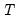observations and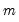potential thresholds (producing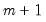regimes). (While we will use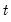to index theobservations, there is nothing in the structure of the model that requires time series data.)
For the observations in regime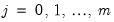we have the linear regression specification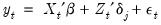(35.1)
Note that the regressors are divided into two groups. The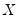variables are those whose parameters do not vary across regimes, while the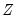variables have coefficients that are regime-specific.
Suppose that there is an observable threshold variable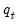and strictly increasing threshold values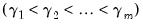such that we are in regime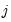if and only if: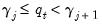where we set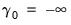and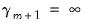. Thus, we are in regimeif the value of the threshold variable is at least as large as the j-th threshold value, but not as large as the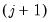-th threshold. (Note that we follow EViews convention by defining the threshold values as the first values of each regime.)
For example, in the single threshold, two regime model, we have: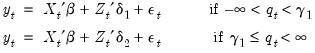(35.2)
Using an indicator function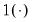which takes the value 1 if the expression is true and 0 otherwise and defining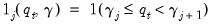, we may combine theindividual regime specifications into a single equation: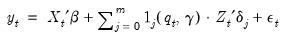(35.3)
The identity of the threshold variableand the regressors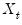and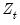will determine the type of TR specification. Ifis the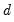-th lagged value of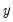, Equation (35.3) is a self-exciting (SE) model with delay; if it’s not a lagged dependent, it's a conventional TR model. If the regressorsandcontain only a constant and lags of the dependent variable, we have an autoregressive (AR) model. Thus, a SETAR model is a threshold regression that combines an autoregressive specification with a lagged dependent threshold variable.
Given the threshold variable and the regression specification in Equation (35.1), we wish to find the coefficients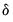and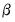, and usually, the threshold values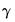. We may also use model selection to identify the threshold variable.
Nonlinear least squares is an natural approach for estimating the parameters of the model. If we define the sum-of-squares objective function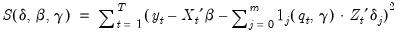(35.4)
and we may obtain threshold regression estimates by minimizing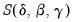with respect to the parameters.
Taking advantage of the fact that for a given, say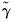, minimization of the concentrated objective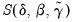is a simple least squares problem, we can view estimation as finding the set of thresholds and corresponding OLS coefficient estimates that minimize the sum-of-squares across all possible sets of-threshold partitions.
This basic estimation setup is well known from the breakpoint testing and regression literature (see, for example, Hansen, 2001 and Perron, 2006), and indeed, by permuting the observation index so that the threshold variable is non-decreasing, one sees that estimation of the threshold and breakpoint models are fundamentally equivalent (Bai and Perron, 2003), In essence, threshold regressions can be thought of as breakpoint least squares regressions with data reordered with respect to the threshold variable. Alternately, breakpoint regressions may be thought of as threshold regressions with time as the threshold variable.
Accordingly, the discussion of breakpoint testing ( “Multiple Breakpoint Tests”) and estimation ( “Least Squares with Breakpoints”) may generally be applied in the current context. We will assume for our purposes that you are familiar with, or can refer to this material, and in the interest of brevity, we will minimize the amount of repetition in our discussion below.